CAT  >  Practice Questions Level 2: Trigonometry - 2

# Practice Questions Level 2: Trigonometry - 2 - Notes | Study Level-wise Practice Questions for CAT Preparation - CAT

 1 Crore+ students have signed up on EduRev. Have you?

This EduRev document offers 15 Multiple Choice Questions (MCQs) from the topic Trigonometry (Level - 2). These questions are of Level - 2 difficulty and will assist you in the preparation of CAT & other MBA exams. You can practice/attempt these CAT Multiple Choice Questions (MCQs) and check the explanations for a better understanding of the topic.

Question for Practice Questions Level 2: Trigonometry - 2
Try yourself:If cosθ = 5/21 sinθ, then the value of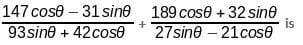Question for Practice Questions Level 2: Trigonometry - 2
Try yourself:The length of the shadow of a vertical pole on level ground increases by 25 metres when the altitude of the sun changes from 60° to 30°. Calculate the height of the pole.

Question for Practice Questions Level 2: Trigonometry - 2
Try yourself:The angles of elevation of a plane flying at a constant altitude of 10000 ft are found to be 60° and 30° at an interval of 1 minute. What is the speed of the plane?

Question for Practice Questions Level 2: Trigonometry - 2
Try yourself:If 4 cos2 A + 2 sin2 A = 3, then what is the value of A, given that A lies in the first quadrant?

Question for Practice Questions Level 2: Trigonometry - 2
Try yourself:AB is a vertical pole. The end A is on the level ground and C is the middle point of AB. P is a point on the level ground. The portion BC subtends an angle β at P. If AP = nAB, then tan  equals

Question for Practice Questions Level 2: Trigonometry - 2
Try yourself:A ladder rests against a vertical wall at an inclination α to the horizontal. Its foot is pulled away from the wall through a distance p, such that its upper end slides a distance q down the wall and then the ladder makes an angle β to the horizontal.
What is the value of P/q?

Question for Practice Questions Level 2: Trigonometry - 2
Try yourself: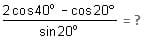Question for Practice Questions Level 2: Trigonometry - 2
Try yourself:If tan α + sin α = m and tan α - sin α = n, then m2 - n2 =

Question for Practice Questions Level 2: Trigonometry - 2
Try yourself:Find the value of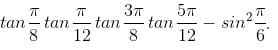Question for Practice Questions Level 2: Trigonometry - 2
Try yourself: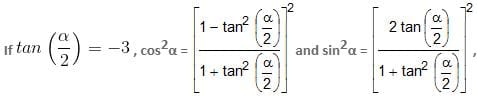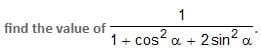Question for Practice Questions Level 2: Trigonometry - 2
Try yourself: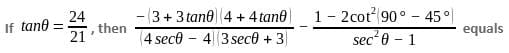Question for Practice Questions Level 2: Trigonometry - 2
Try yourself:A 1.6 m tall observer is 45 meters away from a tower. If the angle of elevation from his eye to the top of the tower is 30°, then the height of the tower in meters is
(Take √3 = 1.732)

Question for Practice Questions Level 2: Trigonometry - 2
Try yourself:The angle of elevation of the top of a tower from a point on the ground at some distance from its base is 60°. The angle of elevation of the top of the tower from a point 20 m above the same point on the ground is 30°. What is the height of the tower?

Question for Practice Questions Level 2: Trigonometry - 2
Try yourself:What is the value of cosθ if n = sinθ - cosθ ?

Question for Practice Questions Level 2: Trigonometry - 2
Try yourself:Two vertical poles of equal height are 120 m apart. On the line joining their bases, A and B are two points. Angle of elevation of the top of one pole from A is 45o and that of the other pole from B is also 45o. If AB = 30 m, then the height of each pole is

The document Practice Questions Level 2: Trigonometry - 2 - Notes | Study Level-wise Practice Questions for CAT Preparation - CAT is a part of the CAT Course Level-wise Practice Questions for CAT Preparation.
All you need of CAT at this link: CAT

## Level-wise Practice Questions for CAT Preparation

277 docs
 Use Code STAYHOME200 and get INR 200 additional OFF

## Level-wise Practice Questions for CAT Preparation

277 docs

### How to Prepare for CAT

Read our guide to prepare for CAT which is created by Toppers & the best Teachers

Track your progress, build streaks, highlight & save important lessons and more!

,

,

,

,

,

,

,

,

,

,

,

,

,

,

,

,

,

,

,

,

,

;